[This article was first published on R Programming – Thomas Bryce Kelly, and kindly contributed to R-bloggers]. (You can report issue about the content on this page here)
Want to share your content on R-bloggers? click here if you have a blog, or here if you don't.

Recently I have been talking a fair bit about my inverse modeling work, so now that it’s summer I finally have time to clean up the code base. Now that the code is working fairly well and offers all of the main features that I can think of, the goal is to get it running quickly and efficiently. Nothing kills motivation quicker than having to wait around for results. There will always be some waiting around, but if I can shave even 10% off of the run time that would be huge.

Since most of the code I’ve written has been boilerplate for the maths which I’ve left to the professionals behind the LIM and limSolve packages, most of the code base doesn’t really call for optimization. Why speed up a bit of code that is only called once and runs for a total of 0.1 seconds?

The majority of the runtime is spent on the maths used in the Monte Carlo sampling, so I’ve focused my energy there. After a preliminary run through the code, most of the function calls and matrix manipulation is direct, efficient and necessary to the functioning of the program. Who ever authored the code originally did a fine job of organizing (and commenting) the code, kudos.

Since R is based on the programming language S, the R interpreter is unable to manage multiple threads or jobs across multiple cores (since S is unable to do so). Therefore all R programs run as a single thread regardless of the computer architecture or processor, and this can be quite painful when you have A) more cores available and B) a computationally intensive task.

While the R interpreter is unable to manage multiple threads, it does not necessarily mean that R programs cannot leverage the extra power of multiple CPUs. But to do so requires calling a script or application written in a language that can run multiple threads. Think of it this way, the R program you’re running can call out to a C++ or Java program that can run the intensive task. At the end of the execution, the helper program can return the answer to your R script and then goes on from there.

This mixing of programing languages is powerful–but just like mixing beer and liquor, it is not without risk and complication. Since my programming experience in this area is minimal and my interest in debugging is low, I’ve shied away from this approach. Your milage will vary.

Just to give a quick example, here is some code that I adapted for use with my code. In effect, it multiplies a matrix and a vector but it does so using C++ and not R.

require(Rcpp)
require(microbenchmark)

#  Source code for our function
func <- 'NumericMatrix mmult( NumericMatrix m , NumericVector v ){
NumericMatrix out(m) ;

for (int j = 0; j < m.ncol(); j++) {
for (int i = 0; i < m.nrow(); i++) {
out(i,j) = m(i,j) * v[j];
}
}
return out ;
}'

#  Make it available
cppFunction( func )

f = function(n) {
for ( i in 1:n) {
mmult(Gg,sol$avg) } } g = function(n) { for ( i in 1:n) { Gg%*%sol$avg
}
}
microbenchmark(f(1000), g(1000))

As you can see here, for the matrices and vectors for which my work deals (i.e.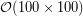) that the C++ function was actually slower to implement than the R equivalent.

expr      min       lq     mean   median       uq      max neval
f(1000) 31.31579 32.60056 34.16907 33.13567 34.47332 71.32896   100
g(1000) 24.15377 26.17468 27.22627 27.04094 27.83810 36.56448   100

So if the code cannot be parallelized, then it stands to reason that the best alternative would be to optimize the execution of the code itself by compiling it properly. To do this, I’ve opted to use the simple method of enabling Just In-Time compilation (JIT) which should significantly speed up the loops and repeated operations in the code. There are a number of example benchmark (eg ) and a good write up (here) by Tal Galili so I’ll spare you the details and move on.

### Vectorization

Vectorization is a cool work to describe a pretty cool concept. In essence, a vector is a conceptual way to view both data and how it is manipulated by software. Unfortunately most beginning programming books introduce the concept of data manipulation in an entirely different way: a way orthogonal to vectorizing. While it does make programing easier to understand at first, it leads to bad habits and poorly performing code.

So what is vectorization in practice. Take for example a basic script that takes a set of numbers and outputs their squares:

> x = c(5,10,3,6,12,1,1,4,13,9)
> for (i in 1:10) {
>     print(x[i]^2)
> }
25 100 9 36 144 1 1 16 169 81

Nothing remarkable here. The code is straightforward and it is easy to understand what the program is doing every step of the way. But here is the equivalent script written in a vectorized form.

> x = c(5,10,3,6,12,1,1,4,13,9)
> print(x^2)
25 100 9 36 144 1 1 16 169 81

Not only does this code do the exact same thing, it runs considerably quicker. Let’s actually time the difference between these two methods. Here we generate 100,000 random numbers and then square them either individually or as a vector. We do this 100 times to get an accurate benchmark.

#Load the package
require(microbenchmark)

## Define our functions
f = function(n) {
x = runif(n)
for (i in 1:n) x[i]^2
}
g = function(n) {
x = runif(n)
x^2
}

## Run the benchmark
n1 = microbenchmark(f(100000), g(100000), times=100)
n1
plot(n1, main="Comparison of Simple and Vector Functions", xlab="Function", ylab="Time")

From the mean execution time of the functions, it is clear that the vector manipulation is much, much quicker! It runs the same operations in nearly 1/10th of the time.

Unit: milliseconds
expr       min        lq      mean    median        uq       max neval
f(1e+05) 59.445756 64.843667 72.041877 68.485962 73.498459 141.86701   100
g(1e+05)  3.993074  6.017984  7.482668  6.196141  6.833197  72.75496   100

Vectorization is a wonderful way to optimize a program, but it can be difficult to implement simply because it’s more difficult to conceptualize.  For my code base, everything that can be vectorized already is (at least everything I can see).

### Misc

After some research it turns out that R has a few somewhat bizarre character quirks. In statements, the curly bracket and parenthesis can be used interchangeably, yet one is actually quicker than the other. To add a cherry to the top,  it is the curly bracket which is actually quicker. From what I have read and whitnessed, the speed difference is smalljust a few percentage pointsand depends on the implementation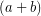is the same asTo illustrate the case, let’s take a look at the (now) classic example from Radford Neal (post):

## Load a required package
require(microbenchmark)

## Generate list of numbers to run operations on
a = runif(20)
b = runif(20)
c = runif(20)

## Definte our functions
f = function(n) for (i in 1:n) d = 1/{a*{b+c}}
g = function(n) for (i in 1:n) d = 1/(a*(b+c))

## Run the benchmark
n1 = microbenchmark(f(100000), g(100000), times=100)
n1
plot(n1, main="Comparison of Parenthesis and Braces", xlab="Function", ylab="Time")

The output of this script clearly shows that the curly bracket (or brace) version of the function runs slightly quicker than the plain parenthesis function (2-3%).

Unit: milliseconds
expr      min       lq     mean   median       uq      max neval
f(1e+05) 160.3369 165.6101 171.7474 168.2779 172.7209 249.8168   100
g(1e+05) 150.7834 166.2364 176.4595 172.1122 176.8393 257.9947   100

While a small speedup like this may not seem like much, depending on the structure of your program it may be worthwhile to check into. Another one of these odds-and-ends that could help boost performance is to pick the best operator for your needs. Take for example a function that returns a square or a square root. There are a number of ways to write either of these functions including,,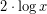,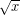,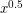and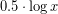; but which way is fastest?

As it turns out for this example is would be best to useandfor these respective operations, but for other cases you’ll just have to test them to find out.

I hope this article has given you some insight into how optimization works and perhaps even how to optimize your own code. Any questions or comments, please feel free to email me at tbk14 at my.fsu.edu.